### maze of circles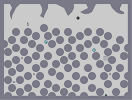Hover over the thumbnail for a full-size version.

Author manaphy author:manaphy awesome circles colab unrated 2008-01-14 2008-01-14 3 more votes required for a rating. \$maze of circles#manaphy#none#000000000:=;<0;<00;<00;HGOH0000;<0:=0:=;<:=;<:1MI000;<:=;<0;<0:=00:=0500000:=00:=0:=;<0;<0;<00000000;<0;<00:=0:=0:=000000;<:=0:=;<00;<0;<0000000:=0;<00:=00:=0:=;0000000;<:=0;<0;<00;<0:0000000:=0;<:=0:=;<:=00LOK@0;<000:=00;<0:=00;<1MI00:=;<000;<:=000;<:=0000000:=;<0:=00;<0:=0;000000;<0:=000;<:=00;<:@00000:=;<0;<0:=0;<0:=0D0000000:=0:=;<00:=00;<1LH000;<00;<0:=;<00;<:=115000:=;<:=;<0:=00:=0;QI000000:=00:=;<0;<0;<:0000000;<0;<00:=0:=0:=00?@0000:=0:=;<0000;<00;HCP000;<0;<0:=0;<0:=00:11A000:=0:=0000:=;<0;<0MI000000;<00;<000:=0:=0000000;<:=00:=;<00000;<000000:=0;<000:=0;<00:=0000000;<:=;<00;<:=;<00H000000:=00:=;<:=00:=001400000000;<0:=00;<00;<11@00000;<:=000;<:=00:=I>P00000:=00;<0:=00;<000000000000;<:=000;<:=0;|5^168,144!3^660,444!6^468,108,3,0,2,1!4^636,570,1!3^204,444!3^132,348!6^276,252,2,1,0,2!11^636,348,84,492!6^564,300,2,0,0,0# me and jackpack worked on this.he made the tileset and i put in the enemies.here is the map data for the tileset: http://numa.notdot.net/map/112352

## Other maps by this author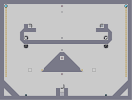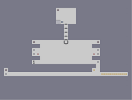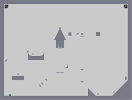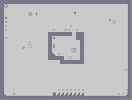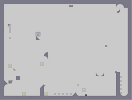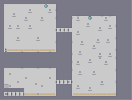the temple of protection missle avoider GET IN THE HOUSE OR YOU'LL CATCH A DEAD you can get out but getting back in... the rocket king the done trap

Pages: (0)

### Yea

Jackpack is right - the level is maybe a bit too easy as it is.
5/5 for the original tileset
3/5 for the placement

how bout making another version of this lvl? ^^

### I agree with jackpack

but i'll give a 3

### 2/5

I liked what you did with my tileset. ;]
The positions of the zap drones were great but the other enemies were in very inefficient positions. There was no point to explore the maze which I had intended for people to do when playing this because there was no gold. I think this could use gold to create a challenge and to have the player explore the maze along with positioning a few enemies in better positions. I gave this a 2 out of 5 because this level could be greatly improved on in those areas.

~JackPack